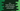# C++ program to find the maximum and minimum of two numbers using cmath# C++ program to find the maximum and minimum of two numbers using cmath :

cmath header provides two functions fmax and fmin to find out the maximum and minimum of two numbers. We can use one if-else block or simply use any one of these functions to find out the max or min between two.

In this blog post, I will show you how to use fmax and fmin functions with examples.

## fmax() Definition :

fmax() is defined as below :

``````double fmax(double x, double y)
float fmax(float x, float y)
long double fmax(long double x, long double y)
``````

Here, x and y are the numbers to compare. It returns the larger number. If you pass NaN to any one of these parameters, it will return the other number.

## fmin() Definition :

fmin() is similar to fmax(). The only difference is that it will return the smaller argument. This method is defined as below :

``````double fmin(double x, double y)
float fmin(float x, float y)
long double fmin(long double x, long double y)
``````

Similar to fmax, if we pass NaN to any one of these parameters, it will return the other.

### Example of fmin() and fmax() :

``````#include <iostream>
#include <cmath>
using namespace std;

int main()
{
double first;
double second;

cout << "Enter the first number : " << endl; cin >> first;

cout << "Enter the second number : " << endl; cin >> second;

cout << "Maximum : " << fmax(first, second) << endl;
cout << "Minimum : " << fmin(first, second) << endl;

return 0;
}
``````

### Sample Output :

``````Enter the first number :
12.33
Enter the second number :
-10.4
Maximum : 12.33
Minimum : -10.4

Enter the first number :
12
Enter the second number :
3
Maximum : 12
Minimum : 3
``````

Here, we are taking the numbers as input from the user. Note that you need to import cmath module to use these methods.